## More Properties of the Fourier Transform

In this lesson, we will cover additional properties of the Fourier Transform. The most useful one is the Convolution Property. It tells us that convolution in time corresponds to multiplication in the frequency domain. Therefore, we can avoid doing convolution by taking Fourier Transforms! In many cases, this will be much more convenient than directly performing the convolution.

### The Convolution Property

The convolution property states that: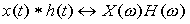Let us show that. To start, let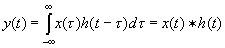Then we can take the Fourier Transform of y(t) and plug in the convolution integral for y(t) (notice how we've marked the integrals with dt and to keep track of them):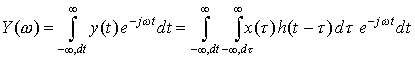Now, let's switch the order of the two integrals (this is valid in all but the most pathological cases):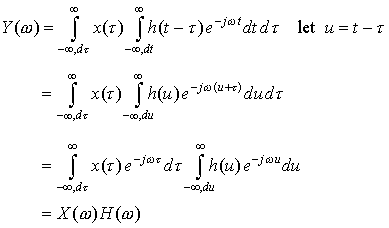Therefore,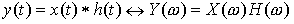We've just shown that the Fourier Transform of the convolution of two functions is simply the product of the Fourier Transforms of the functions. This means that for linear, time-invariant systems, where the input/output relationship is described by a convolution, you can avoid convolution by using Fourier Transforms. This is a very powerful result.

### Multiplication of Signals

Our next property is the Multiplication Property. It states that the Fourier Transform of the product of two signals in time is the convolution of the two Fourier Transforms.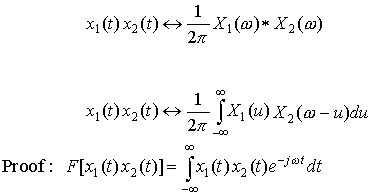Now, write x1 (t) as an inverse Fourier Transform.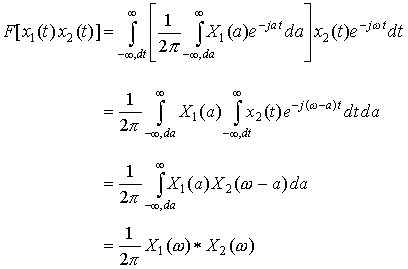Therefore,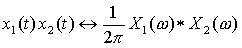Example 1 Find the inverse Fourier Transform of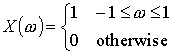Here is a plot of this function: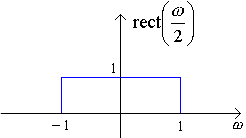Example 2 Find the Fourier Transform of x(t) = sinc2(t) (Hint: use the Multiplication Property).

Example 3 Find the Fourier Transform of y(t) = sinc2(t) * sinc(t). Use the Convolution Property (and the results of Examples 1 and 2) to solve this Example.

### Frequency Shifting or Modulation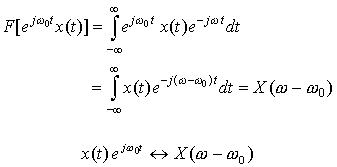This tells us that modulation (such as multiplication in time by a complex exponential, cosine wave, or sine wave) corresponds to a frequency shift in the frequency domain.